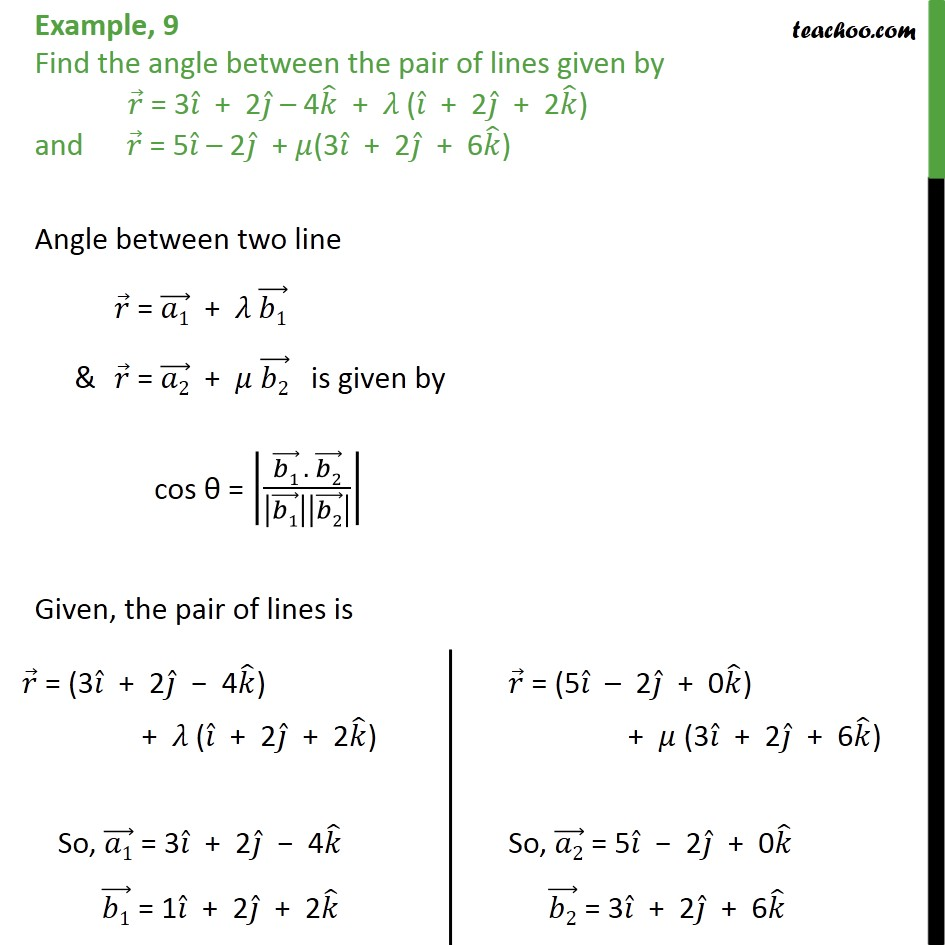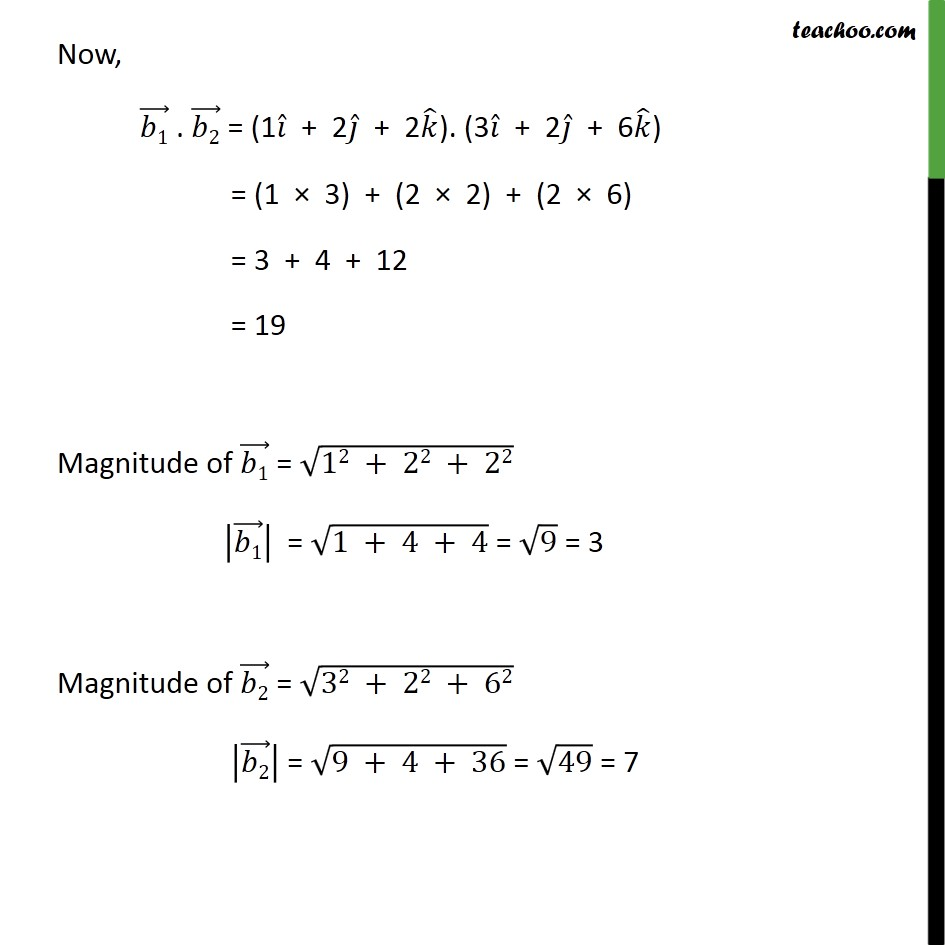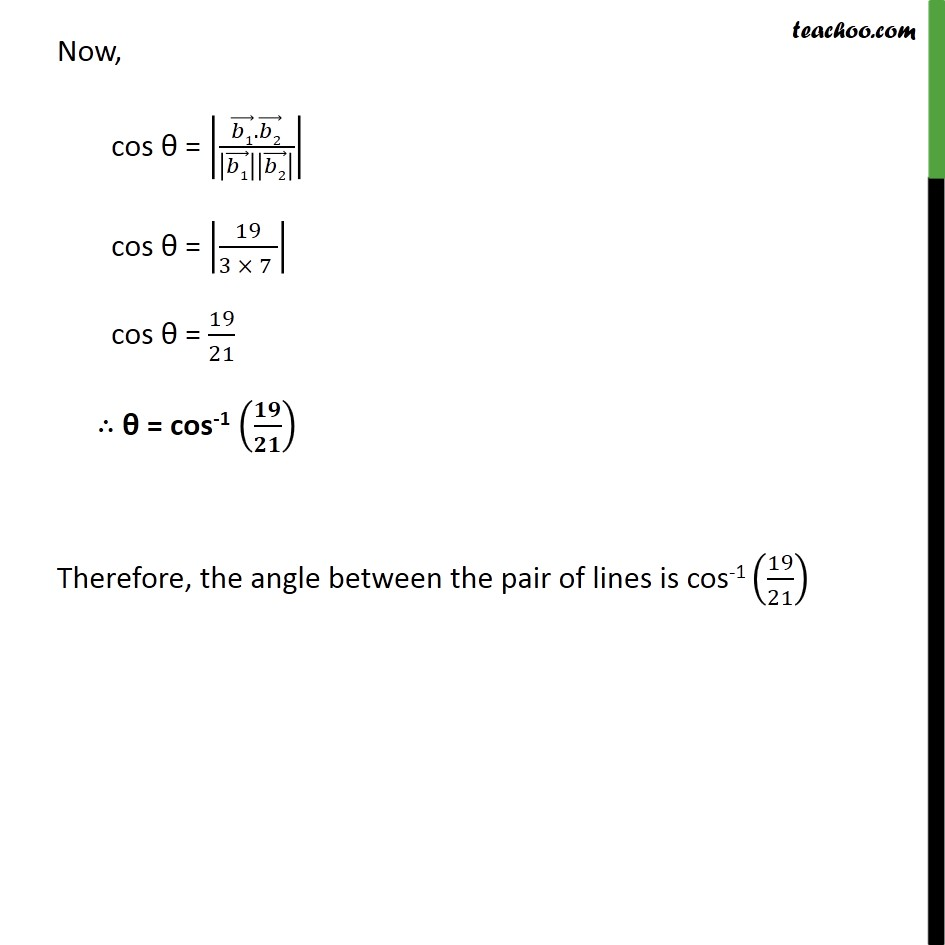1. Chapter 11 Class 12 Three Dimensional Geometry
2. Concept wise
3. Angle between two lines - Vector

Transcript

Example, 9 Find the angle between the pair of lines given by 𝑟﷯ = 3 𝑖﷯ + 2 𝑗﷯ – 4 𝑘﷯ + 𝜆 ( 𝑖﷯ + 2 𝑗﷯ + 2 𝑘﷯) and 𝑟﷯ = 5 𝑖﷯ – 2 𝑗﷯ + 𝜇(3 𝑖﷯ + 2 𝑗﷯ + 6 𝑘﷯) Angle between two line 𝑟﷯ = 𝑎1﷯ + 𝜆 𝑏1﷯ & 𝑟﷯ = 𝑎2﷯ + 𝜇 𝑏2﷯ is given by cos θ = 𝑏1﷯ . 𝑏2﷯﷮ 𝑏1﷯﷯ 𝑏2﷯﷯﷯﷯ Given, the pair of lines is Now, 𝑏1﷯ . 𝑏2﷯ = (1 𝑖﷯ + 2 𝑗﷯ + 2 𝑘﷯). (3 𝑖﷯ + 2 𝑗﷯ + 6 𝑘﷯) = (1 × 3) + (2 × 2) + (2 × 6) = 3 + 4 + 12 = 19 Magnitude of 𝑏1﷯ = ﷮12 + 22 + 22﷯ 𝑏1﷯﷯ = ﷮1 + 4 + 4﷯ = ﷮9﷯ = 3 Magnitude of 𝑏2﷯ = ﷮32 + 22 + 62﷯ 𝑏2﷯﷯ = ﷮9 + 4 + 36﷯ = ﷮49﷯ = 7 Now, cos θ = 𝑏1﷯. 𝑏2﷯﷮ 𝑏1﷯﷯ 𝑏2﷯﷯﷯﷯ cos θ = 19﷮3 × 7 ﷯﷯ cos θ = 19﷮21﷯ ∴ θ = cos-1 𝟏𝟗﷮𝟐𝟏﷯﷯ Therefore, the angle between the pair of lines is cos-1 19﷮21﷯﷯

Angle between two lines - Vector

About the AuthorDavneet Singh
Davneet Singh is a graduate from Indian Institute of Technology, Kanpur. He has been teaching from the past 9 years. He provides courses for Maths and Science at Teachoo.